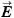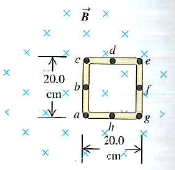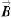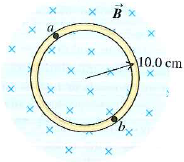Problem

# A uniform, square, conducting loop, 20.0 cm on a side, is placed in the same magnetic fiel...

A uniform, square, conducting loop, 20.0 cm on a side, is placed in the same magnetic field as shown in Exercise 29.30, with side ac along a diameter and with point b at the center of the field (Fig. 29.56). (a) Copy Fig. 29.56, and draw vectors to show the direction and relative magnitude of the induced electric fieldat the lettered points. (b) What is the induced emf in side ac? (c) What is the induced emf in the loop? (d) What is the current in the loop if its resistance is 1.90 Ω? (e) What is the potential difference between points a and c? Which is at higher potential?

Figure 29.56Exercise 29.30

The magnetic fieldat all points within the colored circle shown in Fig. 29.31 has an initial magnitude of 0.750 T. (The circle could represent approximately the space inside a long, thin solenoid.) The magnetic field is directed into the plane of the diagram and is decreasing at the rate of −0.0350 T/s. (a) What is the shape of the field lines of the induced electric field shown in Fig. 29.31, within the colored circle? (b) What are the magnitude and direction of this field at any point on the circular conducting ring with radius 0.100 m? (c) What is the current in the ring if its resistance is 4.00 Ω? (d) What is the emf between points a and b on the ring? (e) If the ring is cut at some point and the ends are separated slightly, what will be the emf between the ends?

Figure 29.31#### Step-by-Step Solution

Request Professional Solution

#### Request Solution!

We need at least 10 more requests to produce the solution.

0 / 10 have requested this problem solution

The more requests, the faster the answer.

All students who have requested the solution will be notified once they are available.